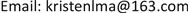1. 引言

2. 理论分析和研究假设

(一) 企业社会责任信息披露与财务绩效。

H1a：积极披露社会责任信息的企业具有更好的财务绩效。

H1b：企业社会责任信息披露对公司财务绩效的影响具有滞后效应。

(二) 高管激励与企业社会责任信息披露

H2a：高管薪酬激励对重污染行业企业披露社会责任信息的质量具有促进作用。

H2b：高管股权激励对重污染行业企业披露社会责任信息的质量具有促进作用。

(三) 企业社会责任信息披露、高管激励和财务绩效

H3a：企业社会责任信息披露水平影响企业对高管的薪酬激励，且高管薪酬激励在企业社会责任信息披露(CSR)对财务绩效的影响中存在中介作用。

H3b：企业社会责任信息披露水平影响企业对高管的股权激励，且高管股权激励在企业社会责任信息披露(CSR)对财务绩效的影响中存在中介作用。

3. 研究设计

(一) 样本选择与数据来源

(二) 变量选择

1) 被解释变量。综合财务绩效(F)，为克服单一指标无法全面反映企业财务绩效的缺陷，借鉴谭丹丹和郑少锋的研究方法  ，本文选取了14个财务指标，利用因子分析法提取6个公因子，建立企业综合财务绩效评价模型，得到综合财务绩效指标F (表1)。

Financial performance indicator

F 1 = 0.211 X 1 + 0.240 X 2 + 0.239 X 3 + 0.238 X 4 + 0.230 X 5 + 0.018 X 6 − 0.077 X 7     + 0.034 X 8 − 0.026 X 9 − 0.037 X 10 − 0.005 X 11 + 0.016 X 12 − 0.096 X 13 − 0.064 X 14

F 2 = − 0.034 X 1 − 0.018 X 2 + 0.010 X 3 − 0.021 X 4 − 0.025 X 5 + 0.007 X 6 + 0.005 X 7     − 0.014 X 8 + 0.508 X 9 + 0.512 X 10 − 0.032 X 11 − 0.016 X 12 + 0.011 X 13 + 0.017 X 14

F 3 = 0.062 X 1 + 0.028 X 2 + 0.026 X 3 − 0.122 X 4 − 0.129 X 5 − 0.047 X 6 + 0.421 X 7     − 0.032 X 8 − 0.007 X 9 − 0.005 X 10 + 0.495 X 11 − 0.038 X 12 − 0.018 X 13 + 0.547 X 14

F 4 = 0.007 X 1 − 0.029 X 2 − 0.027 X 3 − 0.026 X 4 − 0.034 X 5 − 0.005 X 6 − 0.019 X 7     − 0.019 X 8 − 0.004 X 10 − 0.128 X 11 + 0.557 X 12 + 0.729 X 13 + 0.081 X 14

F 5 = 0.169 X 1 + 0.181 X 2 + 0.183 X 3 − 0.313 X 4 − 0.321 X 5 + 0.159 X 6 − 0.291 X 7     + 0.649 X 8 − 0.008 9 − 0.028 X 10 − 0.008 X 11 + 0.071 X 12 − 0.098 X 13 + 0.032 X 14

F 6 = − 0.101 X 1 − 0.085 X 2 − 0.088 X 3 + 0.167 X 4 + 0.171 X 5 + 0.825 X 6 + 0.411 X 7     + 0.163 X 8 + 0.007 9 + 0.017 X 10 − 0.155 X 11 + 0.016 X 12 − 0.016 X 13 + 0.029 X 14

F = ( 27.098 % F 1 + 13.920 % F 2 + 9.971 % F 3 + 8.603 % F 4 + 8.531 % F 5 + 7.280 % F 6 ) / 75.403 %

F = 35.94 % F 1 + 18.461 % F 2 + 13.224 % F 3 + 11.409 % F 4 + 11.314 % F 5 + 9.655 % F 6

2) 解释变量。企业社会责任信息披露(CSR)，以往的相关研究中，学者们对企业社会责任的衡量主要采用自建评分体系、内容分析法、声誉评分法等，这些方法难以避免主观性，因此，本文选用第三方评级机构润灵环球责任评级公司(RKS)发布的企业社会责任报告评级得分作为衡量企业社会责任信息披露水平的指标。为考察不同高管激励模式对企业社会责任信息披露与财务绩效关系的影响，文中所述的高管激励(EI)包括高管货币薪酬激励(PAY)与高管股权激励(STO)，考虑到企业中董事、监事和高管都是影响公司发展战略的重要成员，在何霞、苏晓华(2012)的研究基础上  ，本文将高管货币薪酬激励定义为董事、监事及高管报酬总额的自然对数，并以董事、监事和高管持股比例之和代表高管股权激励。

3) 控制变量。借鉴以往学者的研究(陶文杰等，2012；冯丽艳等，2016)   ，本文在回归模型中加入企业规模(SIZE)、企业性质(STATE)、股权集中度(HHI5)以及披露意愿(VOLC)作为控制变量。各个变量的具体定义如表2所示。

Variable definitio

(三) 模型构建

F i , t = a 0 + a 1 CSR i , t + a 2 SIZE i , t − n + a 3 HHI5 i , t + a 4 STATE i , t + a 5 VOLC i , t + ε (1)

CSR i , t = c 0 + c 1 EI i , t + c 2 SIZE i , t + c 3 HHI5 i , t + c 4 STATE i , t + c 5 VOLC i , t + ε (2)

EI i , t = c 0 + c 1 CSR i , t + c 2 SIZE i , t + c 3 HHI5 i , t + c 4 STATE i , t + c 5 VOLC i , t + ε (3)

F i , t = d 0 + d 1 CSR i , t + d 2 EI i , t + d 3 SIZE i , t + d 4 HHI5 i , t + d 5 STATE i , t + d 6 VOLC i , t + ε (4)

4. 实证结果分析

(一) 描述性统计分析

Sample CSR information disclosure statistic

201281.8817.6537.7417.37
201381.7021.7839.3312.28
201487.9524.3740.5911.96
201587.1827.5843.6311.73
201686.6424.0443.0011.77

Descriptive statistic

F830−2.972.760−0.050.47
CSR83017.6587.9540.8637.4412.44
PAY83013.7017.9515.4215.340.75
STO83000.460.0100.04
SIZE83020.1828.5123.2423.151.56
HHI58300.110.980.560.560.18
STATE830010.6910.46
VOLC830010.4000.49

(二) 回归分析

The lagging effect of CSR information disclosure on corporate financial performanc

CSRi,t 0.0010.003*0.005**0.004**0.002
(0.60)(1.68)(2.56)(2.09)(0.66)
SIZEi,t −0.061***−0.054***−0.061***−0.059***−0.054*
(−5.12)(−3.76)(−3.66)(−3.03)(−1.89)
HHI5i,t 0.422***0.251**0.306**0.371**0.504**
(4.23)(2.10)(2.27)(2.29)(2.14)
STATEi,t −0.144***−0.167***−0.157***−0.152***−0.126*
(−4.42)(−4.35)(−3.69)(−3.01)(−1.72)
VOLCi,t −0.125***−0.142***−0.137***−0.086*−0.026
(−4.10)(−3.85)(−3.16)(−1.69)(−0.35)
_cons1.301***1.171***1.249***1.173***1.043*
(5.26)(3.90)(3.54)(2.83)(1.73)
R20.0760.0660.0700.0720.058

Executive incentives test the return of CSR information disclosure and the mediating effect of executive incentive

Fi,t+2 CSRi,tCSRi,tPAYi,t STOi,t Fi,t+2Fi,t+2
CSRi,t 0.005**0.012***0.001*0.0030.004**
(2.56)(3.99)(1.77)(1.39)(2.30)
PAYi,t 2.521***0.183***
(3.99)(7.05)
STOi,t 8.260*0.683***
(1.77)(3.52)
SIZEi,t −0.061***2.527***3.090***0.138***−0.017***−0.087***−0.050***
(−3.66)(6.50)(8.05)(4.98)(−4.42)(−5.28)(−2.94)
HHI5i,t 0.306**20.802***19.584***−0.432*0.077**0.385***0.253*
(2.27)(6.82)(6.28)(−1.94)(2.49)(2.98)(1.89)
STATEi,t −0.157***−0.976−1.319−0.411***−0.090***−0.082*−0.095**
(−3.69)(−0.94)(−1.20)(−5.83)(−9.22)(−1.94)(−2.09)
VOLCi,t −0.137***3.049***2.436**−0.147**0.038***−0.110***−0.163***
(−3.16)(2.99)(2.33)(−2.05)(3.77)(−2.65)(−3.74)
_cons1.249***−67.524***−40.886***12.265***0.404***−0.999**0.974***
(3.54)(−6.11)(−4.84)(20.98)(4.97)(−2.15)(2.72)
R2 0.0700.3150.2970.1830.2820.1560.093
F7.45945.18841.57721.99438.66815.1178.422

(三) 稳健性检验

Robustness tes

TobinQi,t+2 CSRi,tCSRi,tPAYi,t STOi,t TobinQi,t+2TobinQi,t+2
CSR0.014***0.012***0.001*0.011**0.014***
(3.42)(3.99)(1.77)(2.54)(3.33)
PAY2.521***0.306***
(3.99)(5.16)
STO8.260*0.411
(1.77)(0.94)
CSR*PAY−0.467***2.527***3.090***0.138***−0.017***−0.509***−0.460***
(−12.46)(6.50)(8.05)(4.98)(−4.42)(−13.60)(−12.02)
CSR*STO1.121***20.802***19.584***−0.432*0.077**1.261***1.089***
(3.69)(6.82)(6.28)(−1.94)(2.49)(4.24)(3.56)
F2SIZE−0.274*** (−2.88)−0.976 (−0.94)−1.319 (−1.20)−0.411***−0.090***−0.147−0.237**
(−5.83)(−9.22)(−1.53)(−2.30)
HHI5−0.250**3.049***2.436**−0.147**0.038***−0.202**−0.265***
(−2.58)(2.99)(2.33)(−2.05)(3.77)(−2.13)(−2.70)
STATE11.185***−67.524***−40.886***12.265***0.404***7.419***11.017***
(14.18)(−6.11)(−4.84)(20.98)(4.97)(7.00)(13.62)
F2VOLC0.014***0.012***0.001*0.011**0.014***
(3.42)(3.99)(1.77)(2.54)(3.33)
_cons2.521***0.306***
(3.99)(5.16)
r20.2970.3150.2970.1830.2820.3330.298
r2_a0.2890.3080.2900.1740.2750.3250.289
F41.14045.18841.57721.99438.66840.51934.422

5. 结论与启示